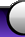HOME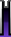NEWSREVIEWSFEATURESFILESFORUMS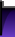The Pronto's IR Code Format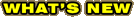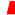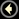[ SEARCH ] [ CONTACT ]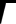ProntoEdit's IR Display Format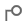How It WorksThe Hex FormatIR Codes Part 1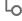IR Codes Part 2By Barry Gordon

...Continued from Page 1.

The ProntoEdit HEX Format.
This discussion is only completely valid for IR transmissions using Pulse Width Modulation. Keep in mind that the sole purpose of the HEX data is to represent a series of ON and OFF times for the IR emitter, and when the IR emitter appear to be solidly ON its is rapidly flashing.

The ProntoEdit HEX format uses a pair of numbers to represent an on/off sequence. We will call this a "Burst Pair" (thanks to AHP). The first digit represents an ON time and the second an Off time.

The question is how much time? What the burst pair really contains is the number of cycles of the carrier for which to turn the light on and off. The carrier frequency therefor acts as the clock (not totally true, but good enough for this discussion). To illustrate the point, let us assume a carrier frequency of 40 kilohertz (that is 40,000 cycles per second). This is a very common IR carrier frequency. One cycle of that carrier takes 1/40000 units of time or 25 microseconds. A "burst pair" of 48,24 would turn the IR emitter on for 48*25 Microseconds, and off for 24*25 microseconds. A "burst Pair" of 24,24 would turn the IR emitter on for 24*25 Microseconds, and off for 24*25 microseconds. Because we are using binary numbers we only have two digits to represent (0,1) as opposed to decimal where we would need 10 unique burst pair patterns to represent the 10 decimal digits. We could for example decide the encoding of a "1" will be represented by having the On period twice as long as the Off period, and a "0" by having them equal. We might choose 48,24 for the "1" and 24,24 for the "0". In fact this is what Sony has done in its IR remotes. [Note: If you work through the numbers you will find that Sony IR signaling uses a sequence of 1200 microseconds of light followed by 600 microseconds of no light to represent a "1"; and a sequence of 600 microseconds of light followed by 600 microseconds of no light to represent a "0"]. In general all IR equipment is forgiving and operates with in a timing tolerance of +/- 10%.

A full IR key code as encoded in the ProntoEdit Hex display contains three discrete parts.

 Preamble Burst Pair Sequence 1 Burst Pair Sequence 2

Either one of the burst pair sequences is optional so we will actually have three different patterns of IR encoding.

 PreamblePreamblePreamble Burst Pair Sequence 1Burst Pair Sequence 1Burst Pair Sequence 2 Burst Pair Sequence 2

The preamble does not contain Burst Pairs but rather four (4) hexadecimal (HEX, base 16) numbers, each of which has a precise meaning. I will only discuss them in the context of Learned IR codes. Each Hex number consists of 4 digits.

1. The first number is always a zero (0000) it indicates that the IR pattern is raw data, which means it was learned.

2. The second number is the frequency of the IR carrier in terms of the Pronto internal clock. The following formula where N represents the decimal value of this hex number will give you the frequency of the carrier in Kilohertz: Frequency = 1000000/(N * .241246)

A Sony remote will usually have a value for N of 103 (this shows as 67 Hex). Doing the arithmetic we have Freq=1000000/(103*. 241246)= 40,244 or approximately 40,000 cycles per second (well within a tolerance of 40,000 +/- 10%)

3. The third number is the number of Burst Pairs in Burst Pair Sequence #1. Each Burst pair consists of two 4 digit Hex numbers representing the On and Off time of that burst (single binary Bit).

4. The fourth number is the number of Burst Pairs in Burst Pair Sequence #2.
Burst Pair Sequence #1 starts at word 5 if it is present and is immediately followed by the digits of Burst Pair Sequence #2 if it is present (word 4>0000). If Sequence #1 is missing (word 3=0000), then Burst Sequence Number 2 starts at word 5.

A Burst Pair Sequence usually looks as follows:

The Lead In Burst pair can be thought of as the hello or wake up burst. It tells the receiver to start listening (or rather looking) very closely as what is coming. It is usually of different timing duration than the Burst Pairs in the data part. Technically it is also used to set the receivers AGC level, a factor related to how much the receiver will amplify the IR light it sees.

The Lead Out burst pair marks the end of the message and usually has a long OFF time period to guarantee that two IR messages can’t be sent too close together. It may actually be incorporated as part of the last data bit if the ON period is what carries the information (that is, the off time is constant in the data portion and the On time varies between two values). Once again, Sony does exactly that.

Remember all data in the IR Hex display is in Hex and to properly interpret these values you must convert them to decimal. Two values should be considered equal if they are within about 10% of each other. They don’t have to be exactly the same.

[Digression to convert a 4 digit Hex "WXYZ" number to decimal, the following formula will work W*4096+X*256+Y*16+Z. W,X,Y,Z represent HEX digits in the range 0-15 where a=10, b=11,c=12,d=13,e=14,f=15. A hex value of 0067 is therefor 16*6+7=103)].

If you understand all of what has been discussed so far you have based the basics of Binary Signaling 101. Go take a breather.

Before we look at some actual IR codes and their detailed formats, we should understand why there might be two burst sequences in the code and not always just one. The first burst sequence is the Once sequence. It is sent if you tap the button on the Pronto which has learned this code. The second burst sequence is called the Repeat sequence. It is sent repeatedly as long as you hold the key on the Pronto down. If you have used an IR remote you already know that all buttons do not "repeat". The two sequences do not have to be the same. In many cases they are, in others they are not. This is generally manufacturer dependent.

###### Continue to page 3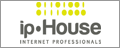©1998-2023, Daniel Tonks. All rights reserved.  Contact | Advertise Home | News | Reviews | Features | Files | Forums | Search